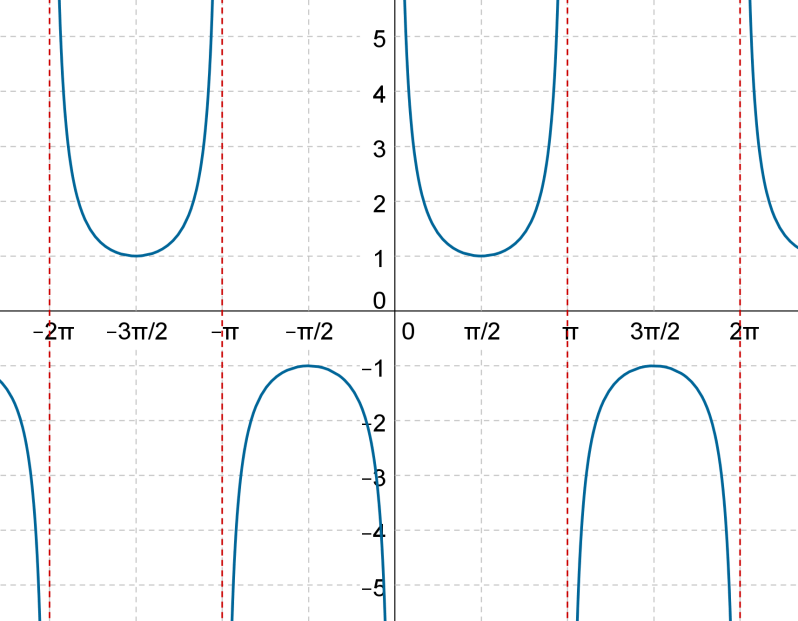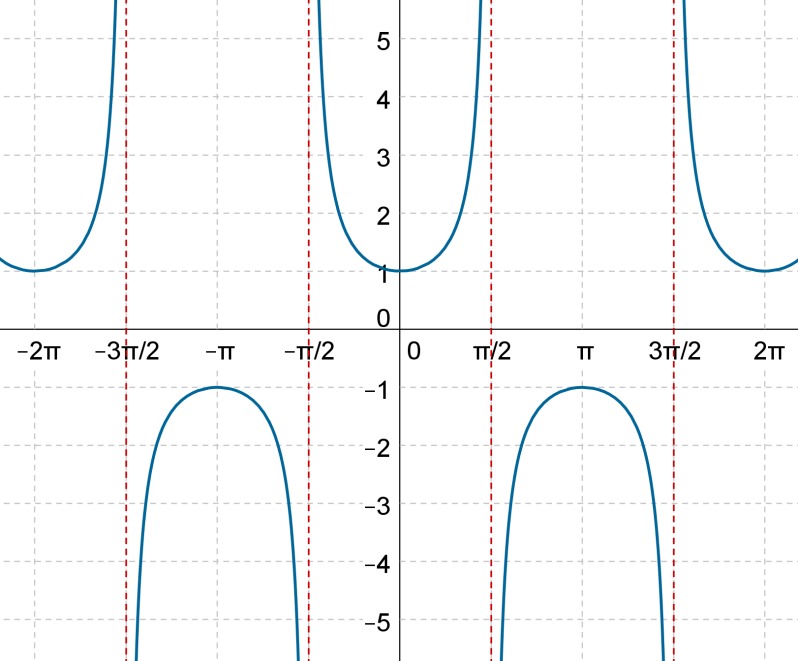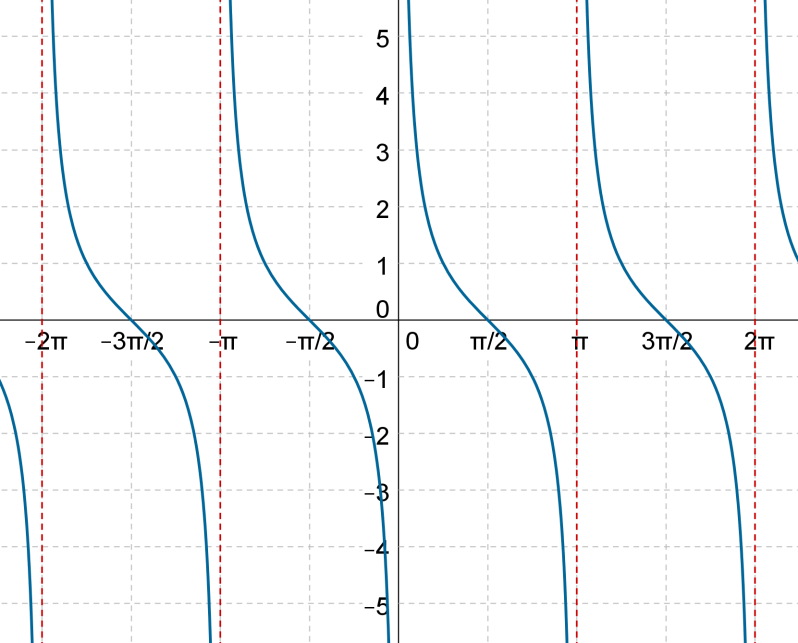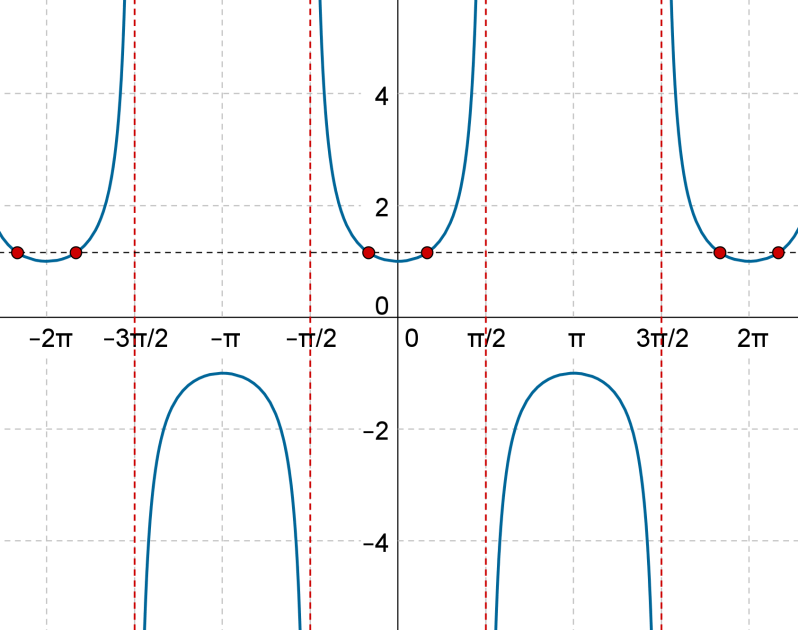Cosecant, Secant and Cotangent Functions

Cosecant, Secant and Cotangent Functions

Introduction:

Our basic three trigonometric functions are sine function, cosine function, and tangent function. The three functions that we are talking about are defined as the reciprocals of our basic three functions. So, we have cosecant which is the reciprocal of sine, secant which is the reciprocal of cosine, and cotangent is the reciprocal of the tangent function. Please go through trigonometric ratios and basic properties of trigonometric ratios to understand the reciprocal relations in a better way.

The simplest way to understand the graphs of these functions is to use the unit circle. In trigonometry, a unit circle is the circle of radius one centered at the origin (0,0) in the Cartesian coordinate system.

Graphs of these trigonometric functions:Think: What will be the graph of these trigonometric functions look like? To understand the procedure followed to plot the graphs of the trigonometric functions, please go through sine function, cosine function, and tangent function.

For your reference, graphs are shown below:

$$f\left( x \right) = {\mathop{\rm cosec}\nolimits}\, x$$Remarks:

• Since $$\sin x = 0$$ for $$x = n\pi$$, the domain of the $${\mathop{\rm cosec}\nolimits} x$$ function is $$\mathbb{R}\ - \left\{ {n\pi } \right\}$$ , where $$n$$ lies in the integer set.

• The magnitude of the sine function does not exceed unity. The magnitude of the cosecant function does not go below unity. From the graph, it is evident that the range of the $${\mathop{\rm cosec}\nolimits} x$$ function is $$\left( { - \infty , - 1} \right] \cup \left[ {1,\infty } \right)$$.

• The cycle length of this function (the period after which it starts repeating) is $$2\pi$$. Thus, for every value of $$x$$ in the domain, we can say that $${\mathop{\rm cosec}\nolimits} \left( {x + 2\pi } \right) = {\mathop{\rm cosec}\nolimits}\, x$$.

$$f\left( x \right) = \sec x$$Remarks:

• To calculate the domain of the secant function, we need to exclude all those values from the real set for which the cosine function is 0. Thus, the domain of the $$\sec x$$ function is $$\mathbb{R}\ - \left\{ {\left( {2n + 1} \right)\frac{\pi }{2}} \right\}$$, where $$n$$ lies in the set of integers.

• As in the case of the cosecant function, the range of the secant function is $$\left( { - \infty , - 1} \right] \cup \left[ {1,\infty } \right)$$.

• The cycle of this function is also $$2\pi$$. Thus, for every $$x$$ (in the domain), we can say that $$sec\left( {x + 2\pi } \right) = secx$$.

$$f\left( x \right) = \cot x$$Remarks:

• From the graph, it is clear that the multiples of $$\pi$$ do not lie in the domain of this function. Thus, the domain of the $$\cot x$$ function is $$\mathbb{R}\ - \left\{ {n\pi } \right\}$$.

• Like the tan function, the range of the cot function is $$\mathbb{R}$$.

• The cycle length of the cot function, like the tan function, is $$\pi$$. Thus, for every value of $$x$$ in its domain, we can say that $$\cot \left( {x + 2\pi } \right) = \cot x$$.

Solved Examples:

Example 1: Find the domain of $$f\left( x \right) = \frac{1}{{{\text{cosec }}x - 2}}$$.

Solution: First of all, the $${\mathop{\rm cosec}\nolimits} x$$ should itself be well-defined. This means that x cannot be an integral multiple of $$\pi$$. Also, we have to exclude those values of $$x$$ for which $${\mathop{\rm cosec}\nolimits} x$$ equals 2. These values are:

$x = 2n\pi + \frac{\pi }{6},\,\,\,2n\pi + \frac{{5\pi }}{6},\,\,\,n \in \mathbb{Z}$

You are urged to work this out on your own. Thus, the domain of the function is

$\boxed{D = \mathbb{R}\ - \left\{ {n\pi ,2n\pi + \frac{\pi }{6},\,\,\,2n\pi + \frac{{5\pi }}{6}} \right\},\,\,n \in \mathbb{Z}}$

Example 2: Find all values of $$x$$ such that $$\sec x = \frac{2}{{\sqrt 3 }}$$.

Solution: The following figure shows the intersection points of $$y = \sec x$$ and $$y = \frac{2}{{\sqrt 3 }}$$:The two points of intersection around $$x = 0$$ are

$x = \frac{\pi }{6},\,\,\,x = - \frac{\pi }{6}$

Thus, we can write the general solution set as

$\boxed{x = 2n\pi \pm \frac{\pi }{6},\,\,\,n \in \mathbb{Z}}$

Note: We could also have solved this equation by instead solving $$\cos x = \frac{{\sqrt 3 }}{2}$$.Challenge: Find all values of $$x$$ such that $$\cot x = 1$$.

Tip: Use a similar approach as in example-2.

Trigonometry
Trigonometry
Grade 9 | Questions Set 1
Trigonometry
Grade 10 | Questions Set 1
Trigonometry
More Important Topics
Numbers
Algebra
Geometry
Measurement
Money
Data
Trigonometry
Calculus
More Important Topics
Numbers
Algebra
Geometry
Measurement
Money
Data
Trigonometry
Calculus
Learn from the best math teachers and top your exams

• Live one on one classroom and doubt clearing
• Practice worksheets in and after class for conceptual clarity
• Personalized curriculum to keep up with school